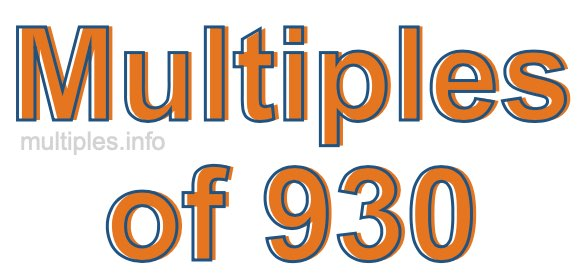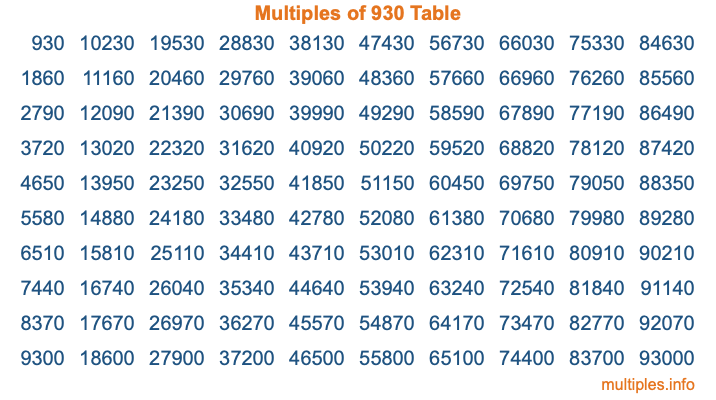Multiples of 930Welcome to the Multiples of 930 page. Here we will first teach you everything you will ever need to know about the multiples of 930, and then give you a study guide summary of everything we taught you to make sure you remember it all. Use this page to look up facts and learn information about the multiples of 930. This page will make you a multiples of nine hundred thirty expert!

Definition of Multiples of 930
Multiples of 930 are all the numbers that when divided by 930 equal an integer. Each of the multiples of 930 are called a multiple. A multiple of 930 is created by multiplying 930 by an integer.

Therefore, to create a list of multiples of 930, you start with 1 multiplied by 930, then 2 multiplied by 930, then 3 multiplied by 930, and so on for as long as you want. Thus, the list of the first five multiples of 930 is 930, 1860, 2790, 3720, and 4650. To see a larger list of multiples of 930, see the printable image of Multiples of 930 further down on this page. We also have a category where you can choose any nth multiple of 930.

Multiples of 930 Checker
The Multiples of 930 Checker below checks to see if any number of your choice is a multiple of 930. In other words, it checks to see if there is any number (integer) that when multiplied by 930 will equal your number. To do that, we divide your number by 930. If the the quotient is an integer, then your number is a multiple of 930.

Is  a multiple of 930?

Least Common Multiple of 930 and ...
A Least Common Multiple (LCM) is the lowest multiple that two or more numbers have in common. This is also called the smallest common multiple or lowest common multiple and is useful to know when you are adding our subtracting fractions. Enter one or more numbers below (930 is already entered) to find the LCM.

Check out our LCM Calculator if you need more details about the Least Common Multiple or if you need the LCM for different numbers for adding and subtraction fractions.

nth Multiple of 930
As we stated above, 930 is the first multiple of 930, 1860 is the second multiple of 930, 2790 is the third multiple of 930, and so on. Enter a number below to find the nth multiple of 930.

th multiple of 930

Multiples of 930 vs Factors of 930
930 is a multiple of 930 and a factor of 930, but that is where the similarities end. All postive multiples of 930 are 930 or greater than 930. All positive factors of 930 are 930 or less than 930.

Below is the beginning list of multiples of 930 and the factors of 930 so you can compare:

Multiples of 930: 930, 1860, 2790, 3720, 4650, etc.

Factors of 930: 1, 2, 3, 5, 6, 10, 15, 30, 31, 62, 93, 155, 186, 310, 465, 930

As you can see, the multiples of 930 are all the numbers that you can divide by 930 to get a whole number. The factors of 930, on the other hand, are all the whole numbers that you can multiply by another whole number to get 930.

It's also interesting to note that if a number (x) is a factor of 930, then 930 will also be a multiple of that number (x).

Multiples of 930 vs Divisors of 930
The divisors of 930 are all the integers that 930 can be divided by evenly. Below is a list of the divisors of 930.

Divisors of 930: 1, 2, 3, 5, 6, 10, 15, 30, 31, 62, 93, 155, 186, 310, 465, 930

The interesting thing to note here is that if you take any multiple of 930 and divide it by a divisor of 930, you will see that the quotient is an integer.

Multiples of 930 Table
Below is an image of the first 100 multiples of 930 in a table. The table is in chronological order, column by column. The first column has the first ten multiples of 930, the second column has the next ten multiples of 930, and so on.The Multiples of 930 Table is also referred to as the 930 Times Table or Times Table of 930. You are welcome to print out our table for your studies.

Negative Multiples of 930
Although not often discussed or needed in math, it is worth mentioning that you can make a list of negative multiples of 930 by multiplying 930 by -1, then by -2, then by -3, and so on, to get the following list of negative multiples of 930:

-930, -1860, -2790, -3720, -4650, etc.

Multiples of 930 Summary
Below is a summary of important Multiples of 930 facts that we have discussed on this page. To retain the knowledge on this page, we recommend that you read through the summary and explain to yourself or a study partner why they hold true.

There are an infinite number of multiples of 930.

A multiple of 930 divided by 930 will equal a whole number.

930 divided by a factor of 930 equals a divisor of 930.

The nth multiple of 930 is n times 930.

The largest factor of 930 is equal to the first positive multiple of 930.

930 is a multiple of every factor of 930.

930 is a multiple of 930.

A multiple of 930 divided by a divisor of 930 equals an integer.

930 divided by a divisor of 930 equals a factor of 930.

Any integer times 930 will equal a multiple of 930.

Multiples of a Number
Here you can get the multiples of another number, all with the same attention to detail as we did for multiples of 930 on this page.

Multiples of
Multiples of 931
Did you find our page about multiples of nine hundred thirty educational? Do you want more knowledge? Check out the multiples of the next number on our list!